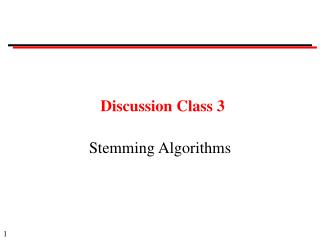DownloadDownload PresentationDiscussion Class 3

# Discussion Class 3

Télécharger la présentation## Discussion Class 3

- - - - - - - - - - - - - - - - - - - - - - - - - - - E N D - - - - - - - - - - - - - - - - - - - - - - - - - - -
##### Presentation Transcript

1. Discussion Class 3 Stemming Algorithms

2. Discussion Classes Format: Question Ask a member of the class to answer Provide opportunity for others to comment When answering: Give your name. Make sure that the TA hears it. Stand up Speak clearly so that all the class can hear

3. Question 1: Conflation methods (a) Define the terms: stem, suffix, prefix, conflation, morpheme (b) Define the terms in the following diagram: Conflation methods Manual Automatic (stemmers) Affix Successor Table n-gram removal variety lookup Longest Simple match removal

4. Question 2: Table look-up (a) What are the advantages and disadvantages of table look-up methods? (b) When would you use table look-up?

5. Question 3: Successor variety methods Hafer and Weiss defined their technique as: Let  be a word of length n, iis a length i prefix of . Let D be the corpus of words. Di is defined as the subset of D containing the terms whose first i letters match i exactly. The successor variety of i, denoted by Si, is then defined as the number of letters that occupy the i+1 st position of words in Di. A test word of length n has n successor varieties Si, Si, ..., Si. Explain this definition, using the word "computation" as an example.

6. Question 4: Successor variety methods With successor variety methods, how do the following methods of segmentation work? (a) cutoff method (b) peak and plateau method (c) complete word method

7. Question 5: n-gram methods (a) Explain the following notation: statistics => st ta at ti is st ti ic cs unique diagrams =>at cs ic is st ta ti statistical => st ta at ti is st ti ic ca al unique diagrams => al at ca ic is st ta ti (b) Calculate the similarity using Dice's coefficient: S = 2C A + B A is the number of unique diagrams in the first term B is the number of unique diagrams in the second term C is the number of shared unique diagrams (c) How would you use this approach for stemming?

8. Question 6: Porter's algorithm (a) What is an iterative, longest match stemmer? (b) How is longest match achieved in the Porter algorithm?

9. Question 7: Porter's algorithm Conditions Suffix Replacement Examples (m > 0) eed ee feed -> feed agreed -> agree (*v*) ed null plastered -> plaster bled -> bled (*v*) ing null motoring -> motor sing -> sing (a) Explain this table (b) How does this table apply to: "exceeding", "ringed"?

10. Question 8: Evaluation (a) What is the overall effectiveness of stemming? (b) Give a possible reason why Stemmer A might be better than Stemmer B on Collection X but worse on Collection Y.